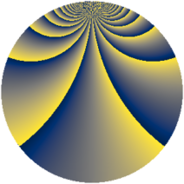# Properties

 Label 2205.2.csLevel $2205$ Weight $2$ Character orbit 2205.cs Rep. character $\chi_{2205}(46,\cdot)$ Character field $\Q(\zeta_{21})$ Dimension $1128$ Sturm bound $672$

# Related objects

## Defining parameters

 Level: $$N$$ $$=$$ $$2205 = 3^{2} \cdot 5 \cdot 7^{2}$$ Weight: $$k$$ $$=$$ $$2$$ Character orbit: $$[\chi]$$ $$=$$ 2205.cs (of order $$21$$ and degree $$12$$) Character conductor: $$\operatorname{cond}(\chi)$$ $$=$$ $$49$$ Character field: $$\Q(\zeta_{21})$$ Sturm bound: $$672$$

## Dimensions

The following table gives the dimensions of various subspaces of $$M_{2}(2205, [\chi])$$.

Total New Old
Modular forms 4128 1128 3000
Cusp forms 3936 1128 2808
Eisenstein series 192 0 192

## Trace form

 $$1128q + 96q^{4} - 2q^{5} - 6q^{7} + O(q^{10})$$ $$1128q + 96q^{4} - 2q^{5} - 6q^{7} + 2q^{10} - 2q^{11} - 16q^{13} + 62q^{14} + 96q^{16} - 12q^{17} + 4q^{19} + 20q^{20} - 16q^{22} + 48q^{23} + 94q^{25} - 10q^{26} + 98q^{28} + 42q^{29} - 8q^{31} + 12q^{34} - 10q^{35} + 102q^{37} + 80q^{38} + 6q^{40} + 16q^{41} + 28q^{43} + 68q^{44} + 66q^{46} + 128q^{47} - 46q^{49} - 196q^{52} + 48q^{53} - 24q^{55} + 206q^{56} - 118q^{58} + 26q^{59} - 60q^{61} + 56q^{62} - 304q^{64} - 6q^{65} - 18q^{67} + 160q^{68} - 14q^{70} - 56q^{71} - 10q^{73} + 2q^{74} + 48q^{76} + 30q^{77} - 10q^{79} + 76q^{80} + 138q^{82} - 30q^{83} + 4q^{85} + 204q^{86} - 34q^{88} + 92q^{89} + 290q^{91} + 60q^{92} + 370q^{94} - 4q^{95} + 136q^{97} + 42q^{98} + O(q^{100})$$

## Decomposition of $$S_{2}^{\mathrm{new}}(2205, [\chi])$$ into newform subspaces

The newforms in this space have not yet been added to the LMFDB.

## Decomposition of $$S_{2}^{\mathrm{old}}(2205, [\chi])$$ into lower level spaces

$$S_{2}^{\mathrm{old}}(2205, [\chi]) \cong$$ $$S_{2}^{\mathrm{new}}(49, [\chi])$$$$^{\oplus 6}$$$$\oplus$$$$S_{2}^{\mathrm{new}}(147, [\chi])$$$$^{\oplus 4}$$$$\oplus$$$$S_{2}^{\mathrm{new}}(245, [\chi])$$$$^{\oplus 3}$$$$\oplus$$$$S_{2}^{\mathrm{new}}(441, [\chi])$$$$^{\oplus 2}$$$$\oplus$$$$S_{2}^{\mathrm{new}}(735, [\chi])$$$$^{\oplus 2}$$